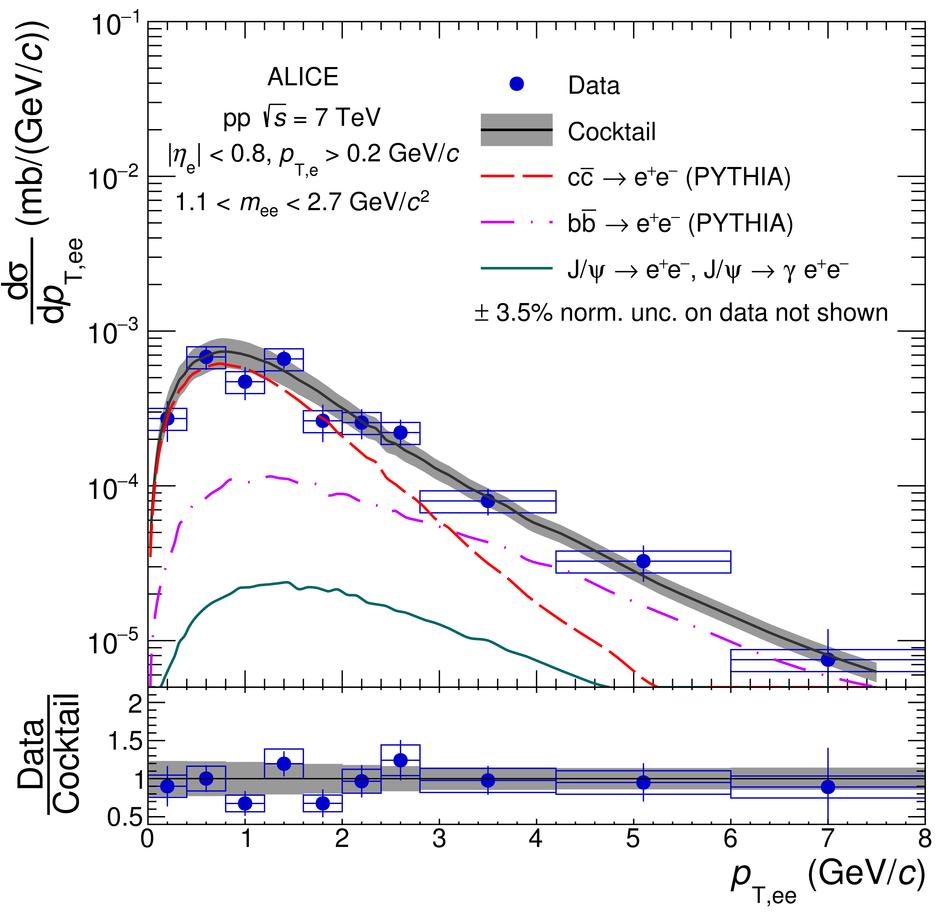Figure 13

 Inclusive \ee cross section in \pp collisions at \roots = 7\,TeV in the ALICE acceptance as a function of \ptee in the mass range 1.1 $<$ \mee $<$ 2.7~GeV/$c^{2}$. The data are compared with the hadronic cocktail, where PYTHIA~ (left) and POWHEG~ (right) are used to calculate the \ccbar and \bbbar contributions. In the bottom panels, the corresponding ratios of data to cocktail as a function of \ptee are shown. Statistical and systematic uncertainties on the data are plotted as vertical bars and boxes, respectively. The total uncertainty of the cocktails is represented as a grey band.